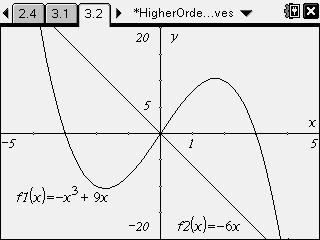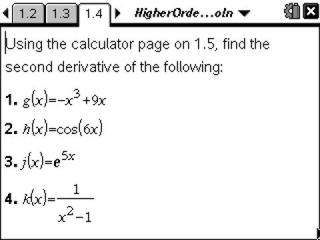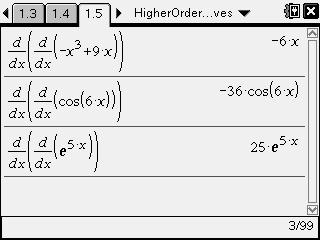# Activities

••• ##### Subject Area

• Math: Calculus: Derivatives

• ##### Author9-12

45 Minutes

• ##### Device
• TI-Nspire™ CAS
• ##### Software

TI-Nspire™ CAS

2.0

## Higher Order Derivatives#### Activity Overview

Students calculate the second derivative of functions, inspect a graph and give the intervals for concave up and concave down and find the point of inflection.

#### Key Steps

•Students will explore the higher derivatives of several functions and use these to identify the concavity and point(s) of inflection.

•Students will use a nested derivative command to find the second derivative of each function.

•Students will graph the function and its second derivative on the same screen to discover the relationship between the function's concavity and second derivative.